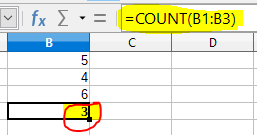#### Question : What will be the result of the formula = COUNT (B1: B3)? Where B1 = 5, B2 = 4, B3 = 6

###### D. 5